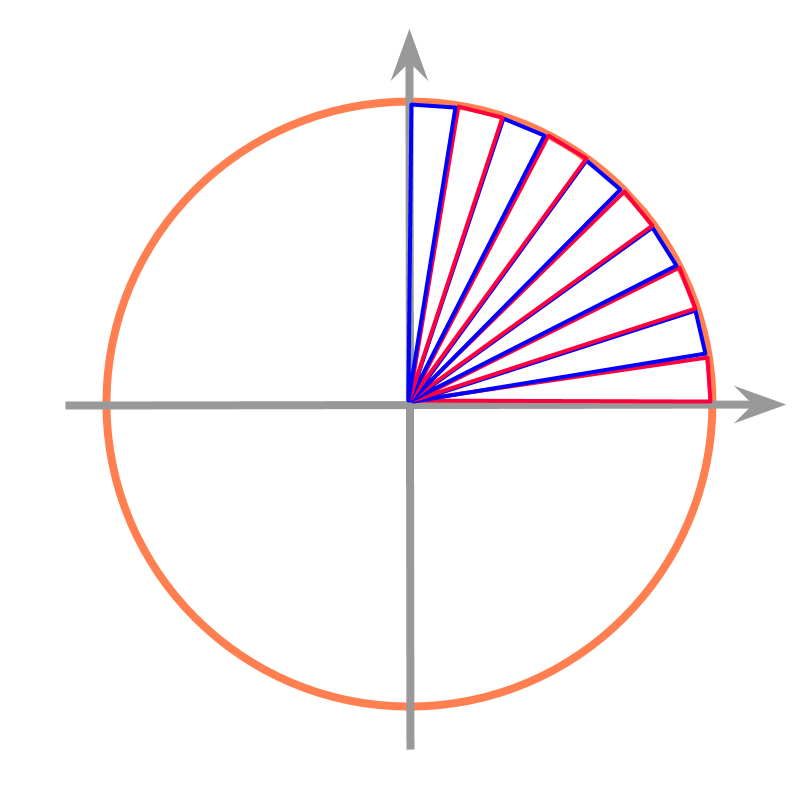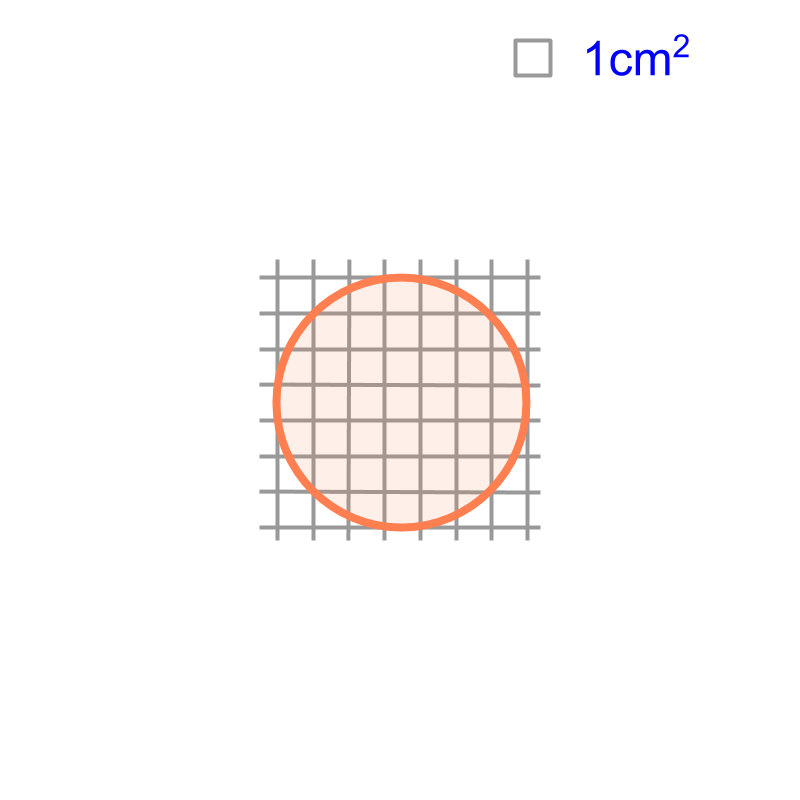maths > mensuration-basics

Circumference and Area of a Circle

what you'll learn...

Overview

Circumference of a Circle: :
Circumference $=2\pi r$$= 2 \pi r$ and $=\pi d$$= \pi d$

$r$$r$ is the radius of the circle

$d$$d$ is the diameter of the circleArea of a Circle :
$=\pi {r}^{2}$$= \pi {r}^{2}$

$r$$r$ is the radius of the circlemeasuring arbitrary length

The word "circumference" means: enclosing boundary.

The word "circumference" is from root words with meaning circum-(around; about) and -ferre(carrying; bearing).

We learned about measurement of objects in real-life, or a line segment of arbitrary length. Some of the learnings are:

•  The length of an object can be an integer value in the unit of measurement. eg: $2$$2$ cm

•  The length of an object need not be exactly an integer value in the unit of measurement. It can be given in smaller unit of length. eg: Length of a pencil is $2cm5mm$$2 c m 5 m m$ or $=25mm$$= 25 m m$ or $=2.5$$= 2.5$ cm

•  The length of an object can be a decimal number in which the decimals do not repeat or do not end. The practical measure of the length is limited by the accuracy of the instrument used in measuring. eg: Length of a pencil is $2.512847\cdots$$2.512847 \cdots$ cm.

Similarly, the circumference of a circle of $1$$1$ cm diameter is a decimal number in which the decimals do not repeat or do not end. That number is approximately $3.14$$3.14$ cm or $\frac{22}{7}$$\frac{22}{7}$ cm.The circumference of a circle of diameter $1$$1$ cm is
$\pi$$\pi$ cm
=approximately $\frac{22}{7}$$\frac{22}{7}$ cm
= approximately $3.14$$3.14$ cm

Circumference of a circle of diameter $1$$1$ cm is represented as $\pi$$\pi$. For a circle of diameter $d$$d$ cm or radius $r$$r$ cm, the circumference is $\pi d$$\pi d$ or $2\pi r$$2 \pi r$ cm.

The circumference of a circle of $7$$7$ cm radius is "$44$$44$ cm"
Approximately $\pi =\frac{22}{7}$$\pi = \frac{22}{7}$

What is the circumference of a circle of $\frac{1}{\pi }$$\frac{1}{\pi}$ cm diameter?
The answer is "$1$$1$ cm"

summary

Circumference of a Circle: :
Circumference $=2\pi r$$= 2 \pi r$ and $=\pi d$$= \pi d$

$r$$r$ is the radius of the circle

$d$$d$ is the diameter of the circlearea is another number

We have learned the following

•  Length of a real-life object or a line can be a decimal number in which the decimals do not end or do not repeat.

•  Circumference of a circle is $\pi$$\pi$ times the diameter.

Similarly, the area of a circle of $1$$1$ cm radius is also a decimal number in which the decimals do not end or repeat. It is calculated that the area of a circle or radius $r$$r$ is $\pi ×r×r$$\pi \times r \times r$ or $=\pi {r}^{2}$$= \pi {r}^{2}$.

More details and derivation of the circumference & area of circle is given in Mensuration High

example

What is the area of a circle of $\frac{1}{\pi }$$\frac{1}{\pi}$ cm radius?
The answer is "$\frac{1}{\pi }c{m}^{2}$$\frac{1}{\pi} c {m}^{2}$".

Area

$=\pi {r}^{2}$$= \pi {r}^{2}$

$=\pi ×\frac{1}{\pi }×\frac{1}{\pi }$$= \pi \times \frac{1}{\pi} \times \frac{1}{\pi}$

$=\frac{1}{\pi }c{m}^{2}$$= \frac{1}{\pi} c {m}^{2}$

summary

Area of a Circle :
$=\pi {r}^{2}$$= \pi {r}^{2}$

$r$$r$ is the radius of the circleOutline

The outline of material to learn "Mensuration basics : Length, Area, & Volume" is as follows.

•  Measuring Basics

→   Introduction to Standards

→   Measuring Length

→   Accurate & Approximate Meaures

→   Measuring Area

→   Measuring Volume

→   Conversion between Units of Measure

•  2D shapes

→   Perimeter of Polygons

→   Area of Square & rectangle

→   Area of Triangle

→   Area of Polygons

→   Perimeter and area of a Circle

→   Perimeter & Area of Quadrilaterals

•  3D shapes

→   Surface Area of Cube, Cuboid, Cylinder

→   Volume of Cube, Cuboid, Cylinder